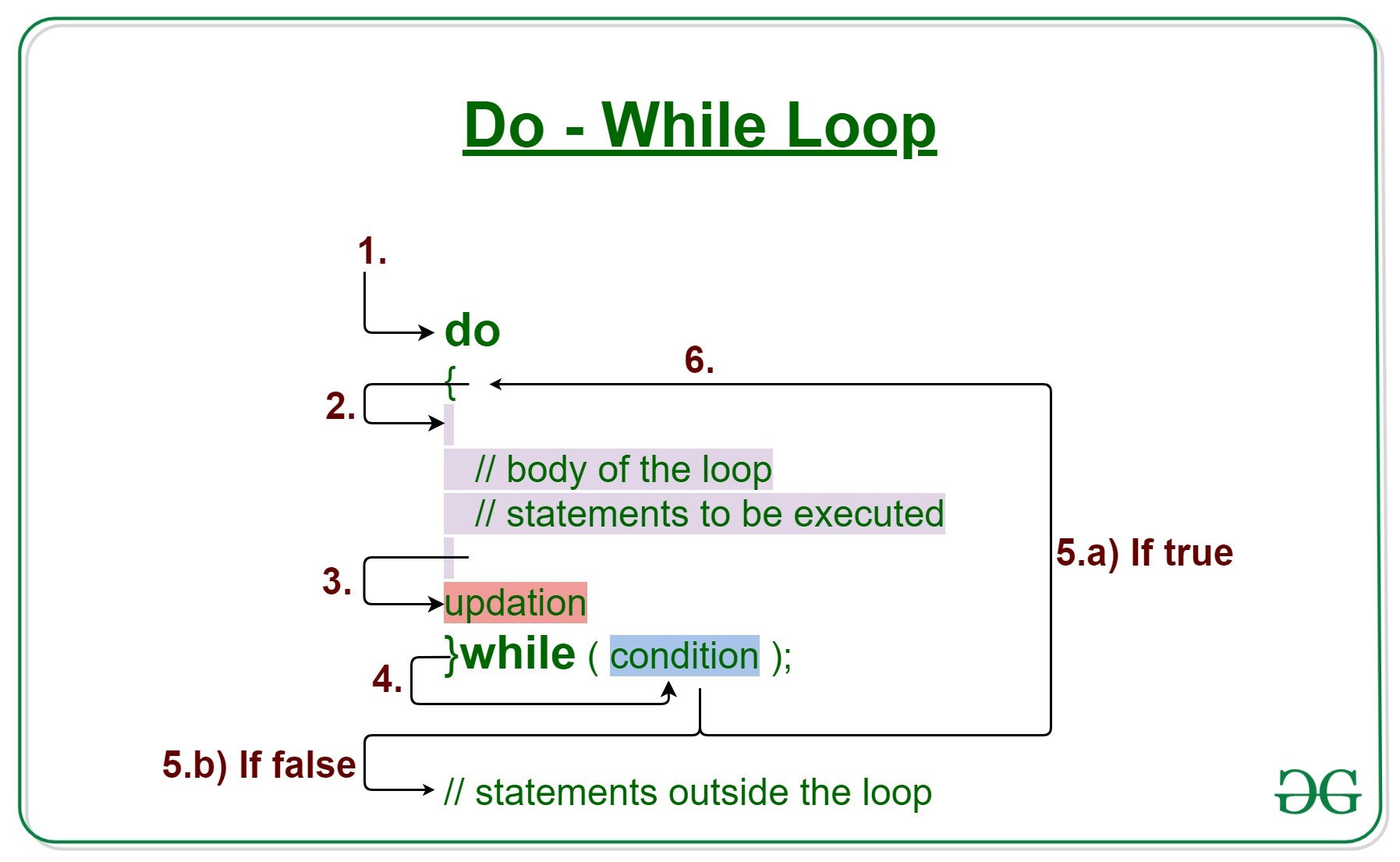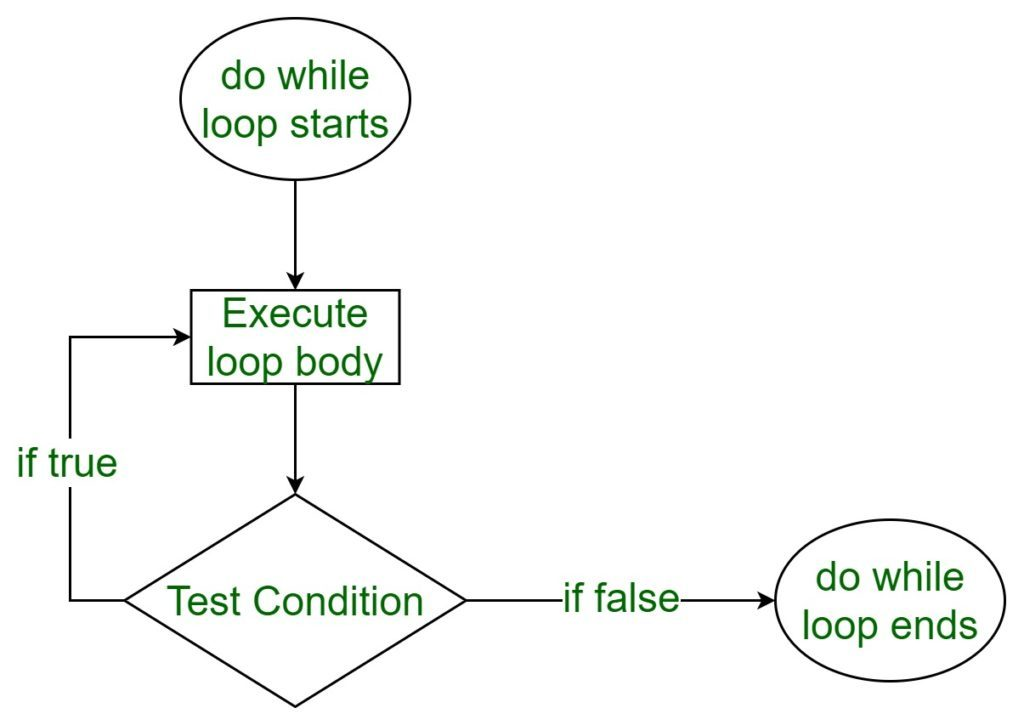# C/C++ do while loop with Examples

• Difficulty Level : Easy
• Last Updated : 22 Jun, 2021

Loops in C/C++ come into use when we need to repeatedly execute a block of statements.
Like while the do-while loop execution is also terminated on the basis of a test condition. The main difference between a do-while loop and while loop is in the do-while loop the condition is tested at the end of the loop body, i.e do-while loop is exit controlled whereas the other two loops are entry controlled loops.
Note: In do-while loop the loop body will execute at least once irrespective of test condition.Take a step-up from those "Hello World" programs. Learn to implement data structures like Heap, Stacks, Linked List and many more! Check out our Data Structures in C course to start learning today.

Syntax:

```do
{
// loop body

update_expression;
}
while (test_expression);```

Note: Notice the semi – colon(“;”) in the end of loop.
The various parts of the do-while loop are:

1. Test Expression: In this expression we have to test the condition. If the condition evaluates to true then we will execute the body of the loop and go to update expression. Otherwise, we will exit from the while loop.
Example:

`i <= 10`
1.
2. Update Expression: After executing the loop body, this expression increments/decrements the loop variable by some value.
Example:

`i++;`
1.

How does a do-While loop executes?

1. Control falls into the do-while loop.
2. The statements inside the body of the loop get executed.
3. Updation takes place.
4. The flow jumps to Condition
5. Condition is tested.
1. If Condition yields true, goto Step 6.
2. If Condition yields false, the flow goes outside the loop
6. Flow goes back to Step 2.

Flow Diagram do-while loop:Example 1: This program will try to print “Hello World” depending on few conditions.

## C

 `// C program to illustrate do-while loop` `#include ` `int` `main()``{``    ``// Initialization expression``    ``int` `i = 2;` `    ``do` `{``        ``// loop body``        ``printf``(``"Hello World\n"``);` `        ``// Update expression``        ``i++;``    ``}``    ``// Test expression``    ``while` `(i < 1);` `    ``return` `0;``}`

## C++

 `// C++ program to illustrate do-while loop` `#include ``using` `namespace` `std;` `int` `main()``{``    ``// Initialization expression``    ``int` `i = 2;` `    ``do` `{``        ``// Loop body``        ``cout << ``"Hello World\n"``;` `        ``// Update expression``        ``i++;` `    ``}``    ``// Test expression``    ``while` `(i < 1);` `    ``return` `0;``}`
Output:
`Hello World`

Dry-Running Example 1:

```1. Program starts.
2. i is initialised to 2.
3. Execution enters the loop
3.a) "Hello World" gets printed 1st time.
3.b) Updation is done. Now i = 2.
4. Condition is checked. 2 < 2 yields false.
5. The flow goes outside the loop.```

Example 2:

## C

 `// C program to illustrate do-while loop` `#include ` `int` `main()``{``    ``// Initialization expression``    ``int` `i = 1;` `    ``do` `{``        ``// Loop body``        ``printf``(``"%d\n"``, i);` `        ``// Update expression``        ``i++;` `    ``}``    ``// Test expression``    ``while` `(i <= 5);` `    ``return` `0;``}`

## C++

 `// C++ program to illustrate do-while loop` `#include ``using` `namespace` `std;` `int` `main()``{``    ``// Initialization expression``    ``int` `i = 1;` `    ``do` `{``        ``// Loop body``        ``cout << i << endl;` `        ``// Update expression``        ``i++;` `    ``}``    ``// Test expression``    ``while` `(i <= 5);` `    ``return` `0;``}`
Output:
```1
2
3
4
5```

Related Articles:

My Personal Notes arrow_drop_up